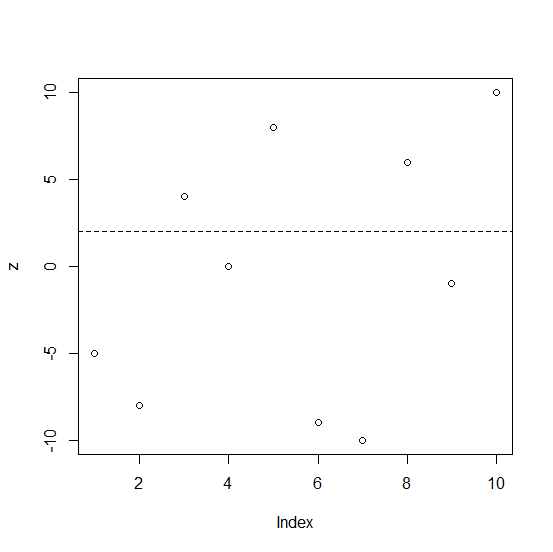# How to create a dashed line that passes through Y = 1 in base R?

Usually, plots are created with solid lines but sometimes we need to use dashed line so that the points can represent a threshold or something critical, the main objective here is to get the attention of the reader on these points. In base R, the plots are created with plot function and we can use abline function with lty =2 to draw dashed lines.

## Example1

Live Demo

x<−−5:5
x
 −5 −4 −3 −2 −1 0 1 2 3 4 5
plot(x)

## Output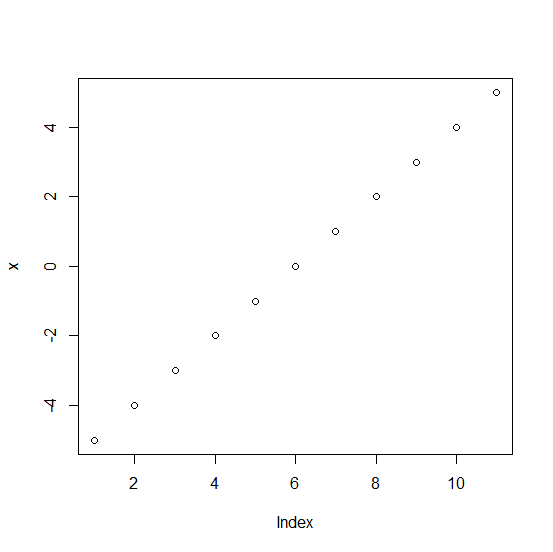## Example

abline(h=2,lty=2)


## Output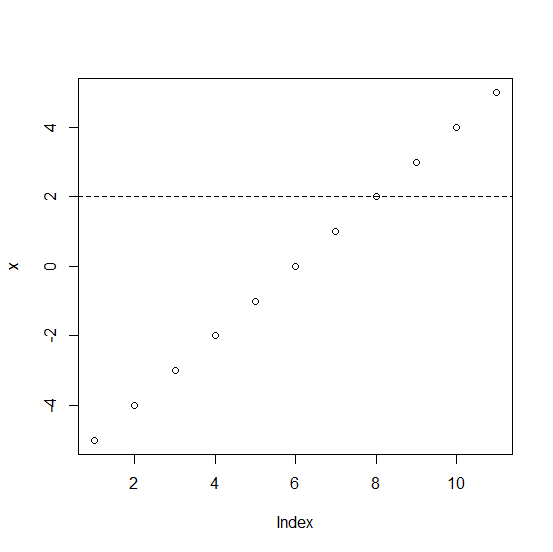## Example

y<−rnorm(10,1,5)
plot(y)

## Output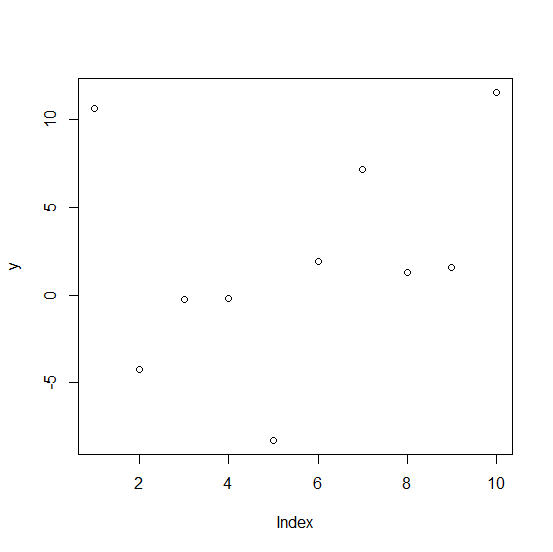## Example

abline(h=2,lty=2)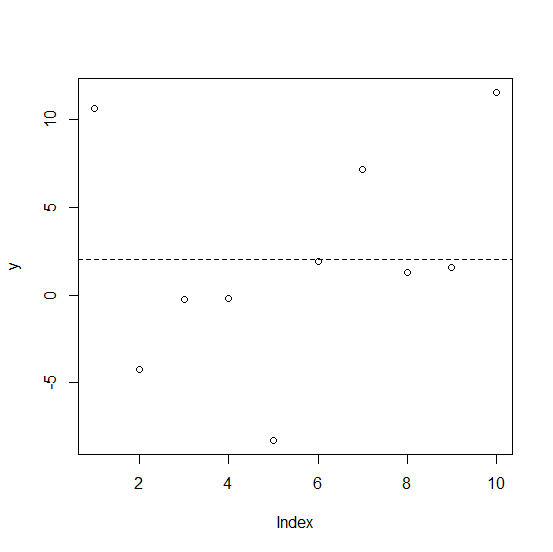## Example3

z<−sample(c(-10:10),10)
z
 −5 −8 4 0 8 −9 −10 6 −1 10
plot(z)

## Output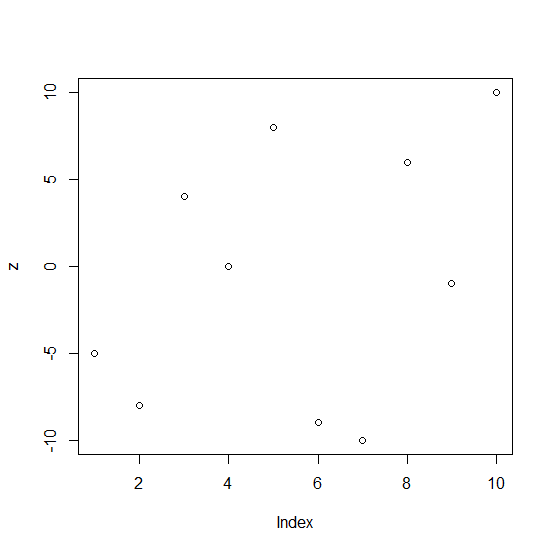## Example

abline(h=2,lty=2)


## Output